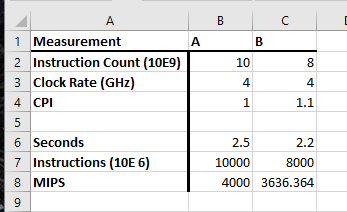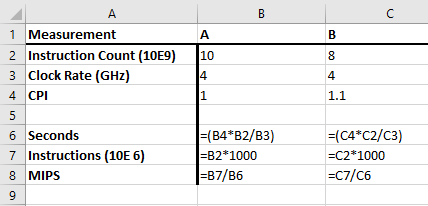$\require{cancel}$

# Some Examples

• Check Yourself, page 53.
Consider the following performance measurements for a program:

MeasurementComputer A Computer B
Instruction Count 10 billion 8 billion
Clock Rate 4 GHz 4GHz
CPI  1.0 1.1

Which computer has the higher MIPS rating?
Which computer is faster?

• To answer part a, we need to know that MIPS is millions of instructions per second.
• For each machine:
• Calculate the total time in seconds.
• Calculate the number of millions of instructions.
• Divide
• Generic Derivations
• For Time in seconds:
• I have: instructions, clock speed, CPI
• I want: seconds
• $\text{seconds} = \frac{\text{seconds}}{ x \text{cycles}} \times \frac{y\text{cycles} }{ \text{instruction}} \times z\text{instructions} = \frac{yz}{x}$
• For millions of instructions
• I have: billions of instructions ($10^{9})$
• I want: millions of instructions ($10^6)$
• Multiply by $10^3$
• I set up a spreadsheet to do the actual computations:
••• Interpretation: Computer A has a faster MIPS rating on the program, it does more instructions in a second than computer B.
• However, computer B finishes the program in less time, so it is faster.
• Please note, I believe that ALL of the above is important for an answer. Simply providing the last two lines, even if your answer is what I have written, is not a satisfactory answer. All portions are required.
• Example 2, Check Yourself page 40:
• A given application written in Java runs 15 seconds on a desktop processor.
A new Java compiler is released that requires only 0.6 as many instructions
as the old compiler.  Unfortunately, it increases the CPI by 1.1.  How fast
can we expect the application to run using the new compiler?  
• Again I want Seconds:
• $\text{seconds} = \frac{\text{seconds}}{ x \text{cycles}} \times \frac{y\text{cycles} }{ \text{instruction}} \times z\text{instructions} = \frac{yz}{x}$
• The problem is we don't know the clock speed or the instruction count.
• So let $i$ be the instruction count of the original program.
• Let $s$ be the clock speed of the original program.
• While not stated, assume the original CPI is 1.
• Original time $15 = t_{old} = \frac{1\times i}{s}$
• We know the new instruction count is $0.6 \times i$
• So the new time is $t_{new} = \frac{0.6i \times 1.1}{s} = 0.6 \times 1.1 \times \frac{i}{s}.$
• But $t_{old} = 15$
• $t_{new} = 0.6 \times 1.1 \times 15 = 9.9 \text{seconds}$